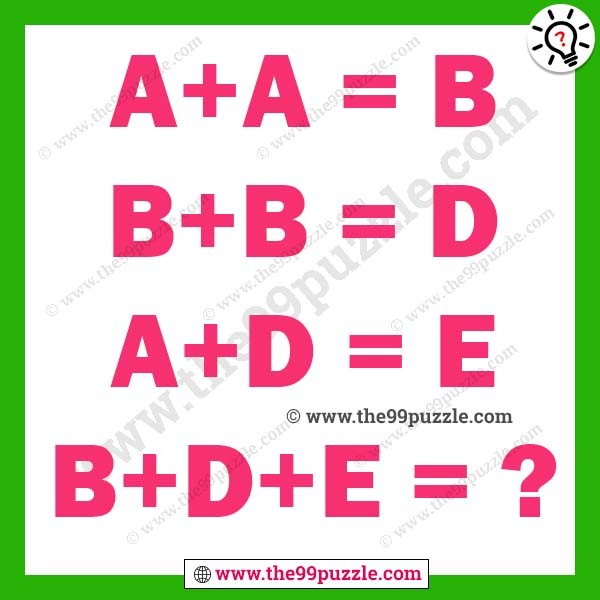# Logical reasoning letter puzzles for adults – Puzz146

In this logical reasoning letter puzzles can solve only genius minds people. You can solve this letter puzzle using your logical thinking. These logical reasoning letter puzzles observe carefully and solve the last equation.

A+A=B

B+B=D

A+D=E

B+D+E=?###### Explanation:

A to Z  are respectively assign the numbers 1 to 26

A=1

B=2

C=3

D=4

E=5

A+A=1+1=2=B

B+B=2+2=4=D

A+D=1+4=5=E

B+D+E=2+4+5=11=K